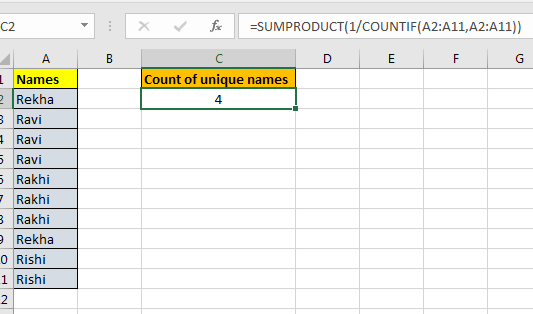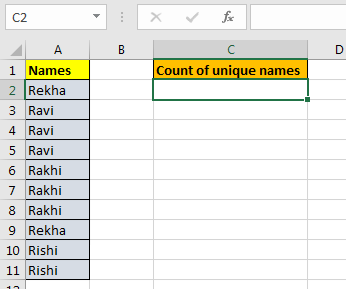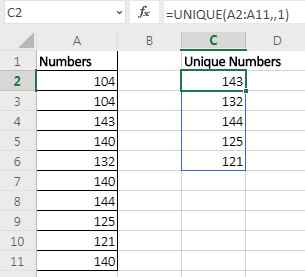# How to count values that appear only once in list in ExcelIn this article, we will learn How to count values that appear only once in a list in Excel.

Scenario:

Sometimes when working with a long list of values, it can be numbers, texts, dates, times, or currency. And we need to find the values that are non repeating in the list. You can either highlight these using Conditional formatting or count these. Non- repeating values are called unique values. For example In a list of groceries there are  Rice, Wheat, Floor and Wheat. Text Values Rice & Floor are unique and non repeating whereas as wheat is repeating. Let's learn how to use this using Common Excel functions for previous version of excel and new function Excel 365 Unique function

For previous versions in Excel

Here we will use the combination of SUMPRODUCT and COUNTIF function. Here COUNTIF function counts the number of values in range. SUMPRODUCT finds the sum of values for only the corresponding 1s.

Formula syntax:

 =SUMPRODUCT (1 / COUNTIF (range , range ) )

range : list of values in given range

For latest Excel version : Excel 365

Unique function Syntax:

 =UNIQUE (array, [by_col], [exactly_once])

Example :

All of these might be confusing to understand. Let's understand how to use the function using an example. Here

Let's say we have a name list. Now this list may contain duplicate values. How can we count unique names only? See below table.Write this Unique Count formula anywhere on the sheet. And you will have a unique count of items in a given range.

 =SUMPRODUCT(1/COUNTIF(A2:A11,A2:A11))

#### Explanation:

First COUNTIF(A2:A11,A2:A11) : This part will return an array of count of each element in the cell. If you select this part of the formula and press F9, you will see the array. If “radha” occurs two times and “Reena” 3 then the array will be. {2,2,3,3,3}.In this example it is {2;3;3;3;3;3;3;2;2;2}.

Now this 1/COUNTIF(A2:A11,A2:A11) : Here we are dividing 1 by each element of the array returned by COUNTIF(A2:A11,A2:A11).  It will again return an array of fractions. If the array returned by countif function is {2,2,3,3,3} then the fraction array will be {0.5,0.5,0.333,0.333,0.333}. If we some them then we will get {1,1}. 1 for each element in the array. For our example it is {0.5;0.333;0.333;0.333;0.333;0.333;0.333;0.5;0.5;0.5}.

Now this SUMPRODUCT(1/COUNTIF(A2:A11,A2:A11)) : It will sum the array provided by 1/COUNTIF(A2:A11,A2:A11), which will be the count of unique values in range.As you can see in the above snapshot the count of unique values is just 4. The above method can only be used to count unique values. The new Excel functions extract all the unique values from the list.

Extract Values that only occur once in the range.

In the above example, we got unique values from the range. If a value was occurring once, twice, or more we got only one stance of it. But if we want to extract values that only occurs once in the range (unique values in range) then the formula will be:

 =UNIQUE(A2:A11,1)Here we set the exactly_once variable to TRUE. This returns the values that are unique in the range/array itself.

Here are all the observational notes using the formula in Excel
Notes :

1. The formula works with numbers, text, date values.
2. Use the same data type to match criteria in criteria list like name in names list, date in date values.
3. Lists as criteria ranges must be of same length, or the formula returns #VALUE! error.
4. Operators like equals to ( = ), less than equal to ( <= ), greater than ( > ) or not equals to ( <> ) can be used in criteria arguments, with numbers only.
5. For example matching time values which have hour value 6. So you can use "06:*:*". Each ( * ) asterisk character is used for the uncertain minutes and seconds.

Hope this article about How to use the ... function in Excel is explanatory. Find more articles on calculating values and related Excel formulas here. If you liked our blogs, share it with your friends on Facebook. And also you can follow us on Twitter and Facebook. We would love to hear from you, do let us know how we can improve, complement or innovate our work and make it better for you. Write to us at info@exceltip.com.

Related Articles :

Excel Formula to Sort Text : To sort text values using formula in excel we simply use the COUNTIF function. Here is the formula

Excel Formula to Extract Unique Values From a List : To find all unique values from a list in Excel 2016 and older versions, we use this simple formula.

UNIQUE Function for Excel 2016 : The Excel 2016 does not provide the UNIQUE function but we can create one. This UDF UNIQUE function returns all unique values from the list.

How To Count Unique Values in Excel With Criteria : To count unique values in excel with criteria we use a combination of functions. The FREQUENCY function is core of this formula

How to use the SUBTOTAL function in Excel : Returns the SUM, COUNT, AVERAGE, STDEV or PRODUCT on applied filtered data in Excel.

Popular Articles :